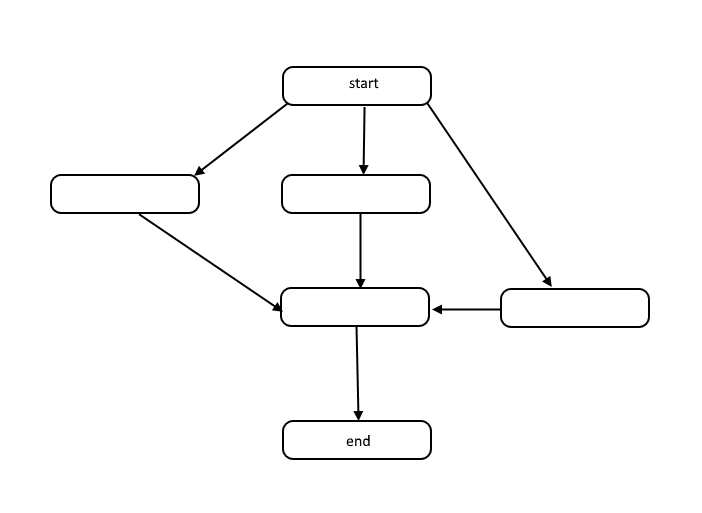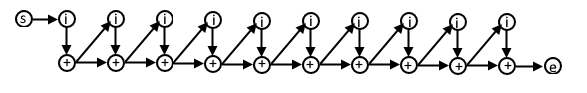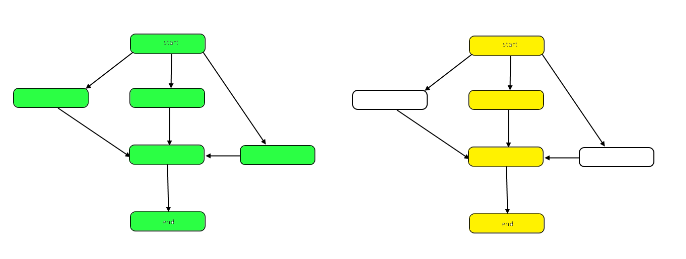# Algorithm Complexity

suggest change

## Remarks

All algorithms are a list of steps to solve a problem. Each step has dependencies on some set of previous steps, or the start of the algorithm. A small problem might look like the following:This structure is called a directed acyclic graph, or DAG for short. The links between each node in the graph represent dependencies in the order of operations, and there are no cycles in the graph.

How do dependencies happen? Take for example the following code:

``````total = 0
for(i = 1; i < 10; i++)
total = total + i``````

In this psuedocode, each iteration of the for loop is dependent on the result from the previous iteration because we are using the value calculated in the previous iteration in this next iteration. The DAG for this code might look like this:If you understand this representation of algorithms, you can use it to understand algorithm complexity in terms of work and span.

# Work

Work is the actual number of operations that need to be executed in order to achieve the goal of the algorithm for a given input size `n`.

# Span

Span is sometimes referred to as the critical path, and is the fewest number of steps an algorithm must make in order to achieve the goal of the algorithm.

The following image highlights the graph to show the differences between work and span on our sample DAG.The work is the number of nodes in the graph as a whole. This is represented by the graph on the left above. The span is the critical path, or longest path from the start to the end. When work can be done in parallel, the yellow highlighted nodes on the right represent span, the fewest number of steps required. When work must be done serially, the span is the same as the work.

Both work and span can be evaluated independently in terms of analysis. The speed of an algorithm is determined by the span. The amount of computational power required is determined by the work.